Moving Solid (MS) Algorithm

In order to simulate landslide problem, we developed the moving solid (MS) algorithm to simulate an obstacle moving in fluids. The moving solid algorithm is constructed based on the internal source function and the partial grid treatment. This algorithm has successfully simulated the 3-D subaerial and submerged landslide problems with LES turbulence mode. Please check the right box to see some examples.

Algorithm overview: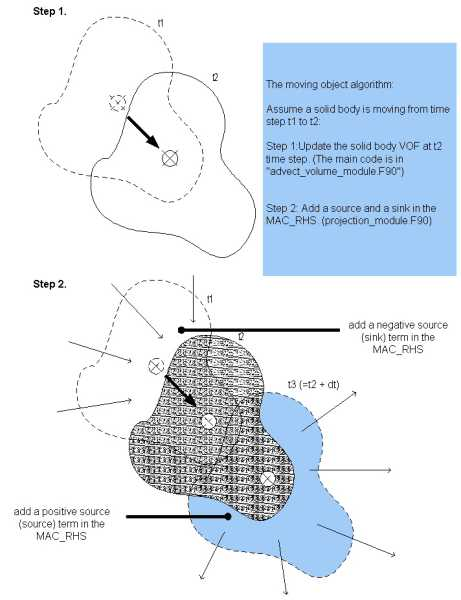A Flying Box

The moving solid algorithm is tested by a simulation of a flying box. The detailed setup can be seen from the figure below. A 0.2mx0.2mx0.2m cubic box is falling down (with a constant speed 0.5 m/s) into a 0.4mx1m water tank with 0.4m still water depth , then moving horizontally with a constant speed 0.5 m/s, and then flying out of the water in a 45-degree angle with a constant speed of 0.707 m/s. Click here to see the animation of how the box interacts with water, and how the waves are generated.

Animation(1,405 KB):

Setup: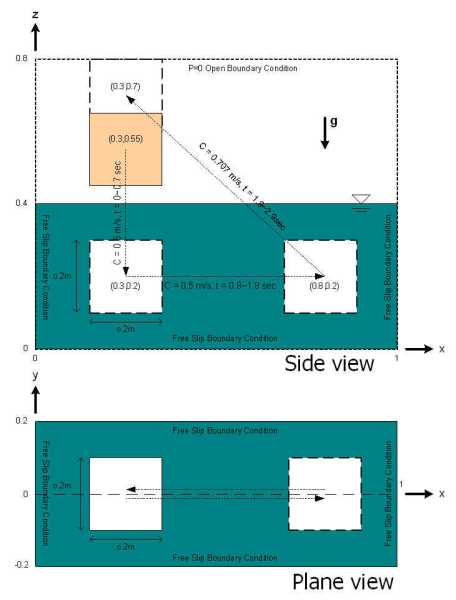1. Good for predicting the complex floating processes.
2. It is able to simulate the movement of a tsunami boulder.
3. Can be used to calculate the pressure field of a high-speed train passing over a underground railroad station.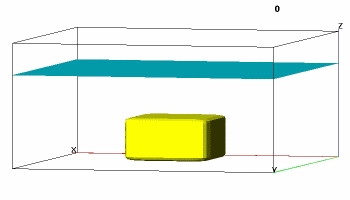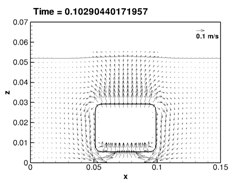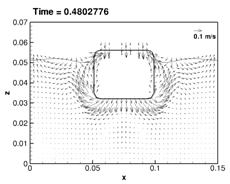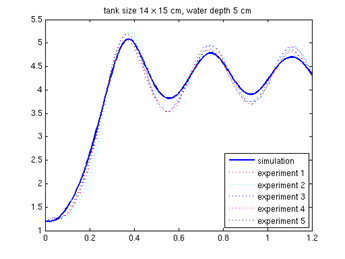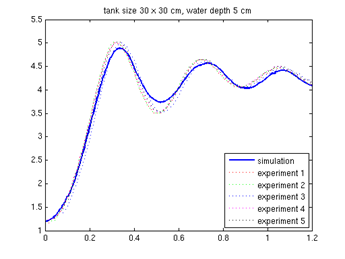Blue: Simulation Dots: Lab data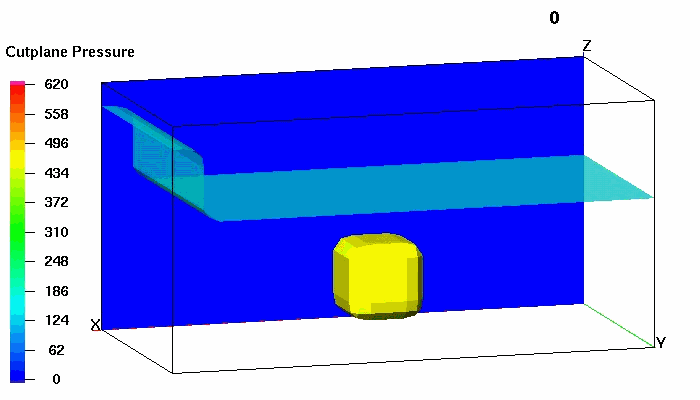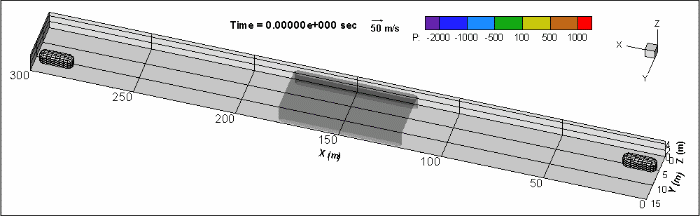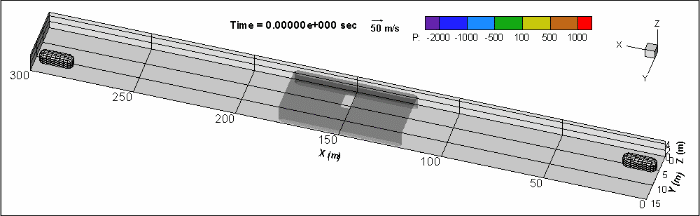Institute of Hydrological and Oceanic Science, National Central UniversityContact: Chuang, Mei-Hui